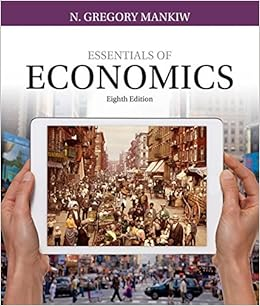# Scenario aggregate consumption equation look at the

• Test Prep
• 83
• 100% (4) 4 out of 4 people found this document helpful

This preview shows page 29 - 32 out of 83 pages.

##### We have textbook solutions for you!
The document you are viewing contains questions related to this textbook.The document you are viewing contains questions related to this textbook.
Chapter 18 / Exercise 5
Essentials of Economics
MankiwExpert Verified
145. (Scenario: Aggregate Consumption Equation) Look at the scenario Aggregate Consumption Equation. If disposable income increases from \$500 to \$800, aggregate consumption will increase by: A) \$0. B) \$200. C) \$240. D) \$440.
146. (Scenario: Aggregate Consumption Equation) Look at the scenario Aggregate Consumption Equation. If all employers announce in September that they guarantee to give all employees a large bonus in December, which of the following equations could represent the new aggregate consumption function?
Page 29
##### We have textbook solutions for you!
The document you are viewing contains questions related to this textbook.The document you are viewing contains questions related to this textbook.
Chapter 18 / Exercise 5
Essentials of Economics
MankiwExpert Verified
147. (Scenario: Aggregate Consumption Equation) Look at the scenario Aggregate Consumption Equation. If the stock market crashes suddenly, which of the following equations could represent the new aggregate consumption function?
148. (Scenario: Aggregate Consumption Equation) Look at the scenario Aggregate Consumption Equation. If housing prices throughout the United States decrease rapidly because of an increase in mortgage foreclosures, which of the following equations could represent the new aggregate consumption function?
149. Planned investment spending depends on all of the following EXCEPT: A) the rate of interest. B) the expected level of real GDP. C) the productive capacity of the economy. D) real GDP.
150. Which of the following is NOT one of the three principal factors upon which planned investment spending depends?
151. All of the following factors determine planned investment spending EXCEPT:
152. Planned investment spending for a given period is:
Page 30
D) always equal to savings. 153. Planned investment spending depends on: A) the market interest rate. B) wealth. C) expected disposable income. D) the life-cycle hypothesis.
154. Most recessions originate from:
155. Investment spending:
•••